# Poynting vector and motor design

Source: Test

Poynting vector - the friendly neighbourhood power balance

Shameful admission here: I only learned about the Poynting vector a few weeks ago. That’s half a year after my freaking PhD! (Not that I think that a doctorate necessarily means anything else besides that you are decently competent in a very, very narrow field).

Of course, I had heard / “learned” of it during my Bachelor’s studies, but that was within the context of radio waves and similar – high-frequency stuff in other words. So, I never thought it would have any bearing to low-frequency magnetics.

Turns out, it does.

Remember the earlier posts about power balance? Get the input power minus losses, and the remainder does something useful. Well, it turns out you can do a similar thing inside a finite element model, too.

### Background, briefly

Recently, I gave a talk (a lecture really, but a talk sounds more impressive) on model order reduction for windings. Specifically, in one of the approaches, a densely meshed slot(s) of a motor is replaced by a single magic element, ridding us of thousands of unknowns to solve. Quite awesome, ainnit?

However, what happens if you want to compute the losses inside the slot?

You could always tear open the magic element and see what makes it tick. But, that’d mean some heavy math and even heavier coding, so I understand if you wanna avoid that.

### Principles, briefly

Luckily, there’s another way. The slot itself has a small time constant.

Why, you might wonder, the windings are in slots and their time constant is quite large, at least when viewed from the motor terminals?

You’d be right to wonder. However, the winding time constant is due to their inductance, which in turn is largely determined by the main magnetic circuit crossing the entire machine. The flux leaving the slot, in other words.

Now, on the other hand, we are only interested in what happens purely inside the slot. Thus, the magnetic path is small and composed of low-permeability materials only, so hence the small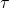.

Where were we? Oh, the small time constant!

Basically, it means that it doesn’t take much to “charge” the magnetic field in the slot. Meaning, if you put 10 millijoules of energy into the slot, it’s very likely you won’t ever be getting more than a few back.

What this means for simulations is this. If you compute the total power being put into the slot – what goes in versus what comes out – you already have a decent estimate for the losses inside. Average it over the brief period and it’s even better. (And of course, average over the entire fundamental period is exact, up to numerical noise and time-stepping errors.)

This, finally, brings us to the main point – how to compute the power going into the slot? Briefly put, it can be divided into two components:

1. Electrical power going into the slot, through the circuit connections. Calculated from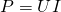.
2. Magnetic power going through the slot/not-a-slot boundary. Calculated with the Poynting vector.

Now, let’s see some details.

Disclaimer: I’m like 95 percent sure I got a minus wrong somewhere, or something. So try to focus on the big picture. You can always sort out the details by doing a test simulation. What doesn’t explode = probably correct.

### Principles, not-so-briefly

Let’s approach this problem from the energy conservation point of view, using the vector potential formulation in 2D. First, we compute the change in the total energy stored in the magnetic field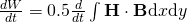.

Now, by remembering that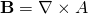and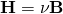, we get after a few simplifications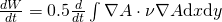.

Next, the time-derivative can be moved inside the integral, after which taking the derivative of the product yields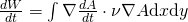Next, we use do some integration by parts and get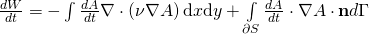.

Then, how to deal with the first term?

Take a look at the basic principles of FEA here, where we wanted that the vector potential satisfies the equation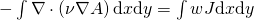with any function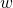. The time-derivative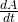certainly counts as “any function”, so we conclude the integral term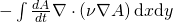must be equal to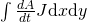.

##### What it means

This all brings us exactly where we want to be. Indeed, once we realize that the time-derivative of the vector potential is the induced electric field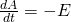,

we can form the following equality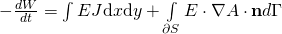.

The left-hand side is the change in the magnetic energy, that we started with. This must equal the power transferred through the electric circuit and lost as heat (first term on the right) plus the power transferred through the boundary.

Finally, once we realize that the normal derivative of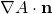corresponds to the tangential component of the magnetic field, we see that the boundary integral is nothing more than an integral of our Poynting vector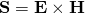.

### Uses

Finally, let’s return to the case of losses inside a reduced slot-model. We deduced that the changes in magnetic energy are small and fast, so the losses inside a slot pretty much equal the power put into it.

Now, we can actually compute this power, too. The first part we get from the electric circuit, by multiplying the voltage(s) over the slot by its current(s). If the problem is solved with the AVI-formulation, both are quite readily available.

The second part, the energy transferred by the magnetic field, we get from the boundary integral derived just moments ago.

Combine the two, and you have the losses.

### Conclusion

The Poynting vector indeed does have its uses even in low-frequency electromagnetics. It can be used to evaluate the energy balance on a local scale. This, in turn, can be used to e.g. compute losses in parts of the problem domain modelled by nonstandard means.

Additionally, it can be used to impose or compute the power flow over the problem boundary. This can be useful for e.g. coupling different problem domains together in a slightly weaker sense than normally. Although the details were not covered in this post, the same principles still apply.

The post Poynting vector and motor design appeared first on Antti Lehikoinen.